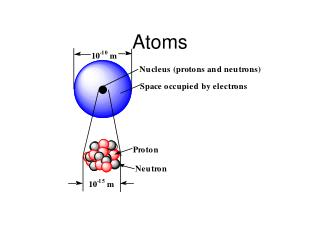DownloadDownload PresentationAtoms

# Atoms

Télécharger la présentation## Atoms

- - - - - - - - - - - - - - - - - - - - - - - - - - - E N D - - - - - - - - - - - - - - - - - - - - - - - - - - -
##### Presentation Transcript

1. Atoms

2. Periodic Table and Electron Configurations • Build-up order given by position on periodic table; row by row. • Elements in same column will have the same outer shell electron configuration.

3. The relation between orbital filling and the periodic table

4. Electron Configuration • Orbitals have definite shapes and orientations in space (insert Fig 2.11 of text) (if it will not all fit on one screen, put part (a) on one screen and part (b) on the next)

5. Orbital occupancy for the first 10 elements, H through Ne.

6. Trends in the Periodic Table Atomic radii of the main-group and transition elements.

7. Trend for atomic radii • Left to right atoms get smaller • Why? • Increase in nuclear charge • More protons and more electrons means greater electrostatic attractions (stronger magnet) • Top to bottom atoms get larger • Why? • Increase in energy levels (You are adding floors to your hotel). Electrons are further from the nucleus

8. Atomic Radius Atomic Radii for Main Group Elements • Atomic radii actually decrease across a row in the periodic table. Due to an increase in the effective nuclear charge. • Within each group (vertical column), the atomic radius tends to increase with the period number.

9. r decreases r increases The Size of Atoms and Ions Radii of neutral atoms The atomic radius of an atom is defined as half the distance between the nuclei in a homonuclear bond. • In general: • - radii decrease across a period because of increasing Z*. • radii increase down a group because of the increasing distance of the electrons from the nucleus. • - increasing distance from the nucleus outweighs effective nuclear charge for atomic radii down a group.

10. Remember that the maximum probability for an orbital moves further away from the nucleus with increasing n. 0.1 nm = 1 Å = 100 pm The “d-block contraction” causes Ga to be about the same size as Al. This is caused by the introduction of the 3d elements which cause a vastly larger Z* for Ga. Bohr model for H; radius(n) = n2a0

11. Trend for Ion Size • Ion is a charged atom. • Metals lose electrons and nonmetals gain electrons to create ions. • Cations are pawsitive (positive) and Anions are negative. • Cations are smaller than their corresponding atom. Why? • Loss of electrons means the positive nucleus has a greater attraction on the remaining electrons • Anions are larger than their corresponding atom. Why? • Gain of electrons means the nucleus has less attraction for the electrons as well as the electrons are repulsing each other causing an increase in the size of the electron clouds

12. Radii of ions This is a “self-consistent” scale based on O-2 = 1.40 (or 1.38) Å. Ionic radii depend on the magnitude of the charge of the ion and its environment. (more later) Positively charged ions are smaller than their neutral analogues because of increased Z*. Negatively charged ions are larger than their neutral analogues because of decreased Z*. Same periodic trends as atomic radii for a given charge

13. Trend for ion size • Decrease across a period then jumps in size at nonmetals and continues to decrease • Increases on the way down a group as you are adding energy levels (electrons are farther from the nucleus)

14. Ionization energy • The energy required to remove an electron

15. First ionization energies of the main-group elements Trends in the Periodic Table

16. Ionization Energy • Ionization energy is a periodic property • In general, it increases across a row. Why? • increasing attraction as the number of protons in the nucleus increases (stronger magnet) • it decreases going down a group. Why? • Outer shell electrons are further from the nucleus so less electrostatic attraction. Nucleus has less pull on them

17. 6) The trend across from left to right is accounted for by a) the increasing nuclear charge.

18. Electronegativity (electron affinity - This is the most important trend to understand for this class. • The tendency for an atom to attract electrons when chemically bonded. • Same trend as ionization energy. • In general, it increases across a row. Why? • increasing attraction as the number of protons in the nucleus increases (stronger magnet) • it decreases going down a group. Why? • Outer shell electrons are further from the nucleus so less electrostatic attraction. Nucleus has less pull on them

19. Trends in three atomic properties See chart in book for summary

20. Check for understanding • Which of the following atoms has the largest atomic radii, ion size, electronegativity, and ionization energy • Na, Mg, K, Ca, S, Cl, Se, Br

21. rVDW decreases rVDW increases van der Waals radii van der Waals radii are established from contact distances between non-bonding atoms in “touching” molecules or atoms VDW radii allow us to determine whether there can be a bonding interaction between two atoms: If the distance between the nuclei is larger than the sum of the VDW radii, then the atoms are probability not bonded.